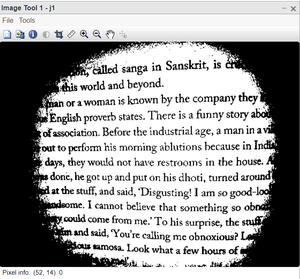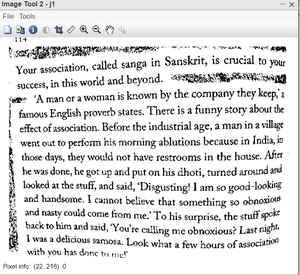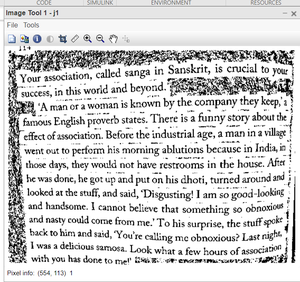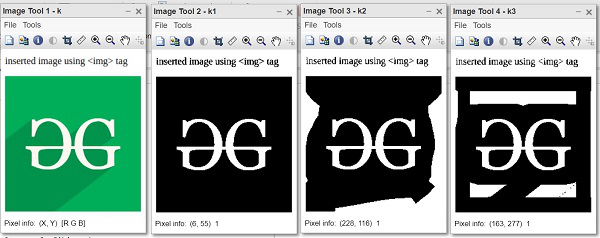Binarization of Digital Images Using Otsu Method in MATLAB

• Difficulty Level : Hard
• Last Updated : 23 Nov, 2021

Binarization is important in digital image processing, mainly in computer vision applications. Thresholding is an efficient technique in binarization. The choice of thresholding technique is crucial in binarization. There are various thresholding algorithms have been proposed to define the optimal threshold value.

Binarization can be used in recognising text and symbols, e.g. document processing. Identifying objects with distinctive silhouettes, e.g. components on a conveyor in a manufacturing plant, and determining the orientation of objects are some other examples of binarization applications. Binarization generally involves two steps including the determination of a gray threshold according to some objective criteria and assigning each pixel to one class of background or foreground. If the intensity of the pixel is greater than the determined threshold then it belongs to the foreground class and otherwise to the background. The main problem in binarization is the choice of thresholding technique.

The thresholding algorithms can be categorized into different classes:

• Histogram shape-based methods
• Clustering-based methods
• Entropy-based methods
•  Object attribute-based methods
•  Spatial methods and local methods are based on the local characteristics of each pixel.

Grayscale image: A grayscale image is the one that has the same value for each channel. Grayscale images have only colours of gray shades, Gray shades are not only black and white. If the image has 8-bit depth. It can have 255 shades of gray between dark and white. Sometimes grayscale images are also called black and white images.

Binary image: Binary images are useful in many image processing applications due to their features. A binary image is produced by quantization of the image gray levels to two values, usually 0 and 1. A binary image has only two shades of colour. All the pixels have either dark or white as colour. The dark shade is represented by 0 and the white shade is represented by 1. The image matrix consists of 0 and 1 only.

Otsu’s method: Otsu’s thresholding technique is a classification-based method which searches for the threshold that minimizes the intra-class variance, defined as a weighted sum of variances of the two classes. It is the most popular method of binarizing a grayscale image. Otsu’s algorithms simply assume that a grayscale image consists of two types of pixels. Foreground and background pixels. It divides all the pixels into two clusters. It minimises the intra-cluster variation by maximising the inter-cluster variance. Finally, it returns a single intensity value which is called a threshold value. This threshold value divides the two clusters of pixels. All pixels of one cluster are assigned intensity value 0 and pixels of the second cluster are assigned value 1. Thus, it binarises the grayscale image.

Steps:

• Convert it into a grayscale image.
• Apply Otsu’s thresholding function.
• Convert grayscale image into a binary image using a threshold.
• Display the binary image.
• Binarize the grayscale image using the local otsu’s method.
• Display the image.

Function Used:

• imread ( ) inbuilt function is used to read the image.
• imtool( ) inbuilt function is used to display image.
• rgb2gray( ) inbuilt function is used to convert RGB image into grayscale.
• graythresh( ) inbuilt function is used to find Otsu’s threshold.
• im2bw( ) inbuilt function is used to convert grayscale into binary image.
• nlfilter( ) inbuilt function is used to iterate the function over distinct blocks of [m n] size.

Example:

Matlab

 % MATLAB code for% OTSU binarisation%read the colored image.k=imread("apple.jpeg");  %display the image.imtool(k,[]);  %convert into grayscale image.k=rgb2gray(k);  %calculate threshold using Otsu's method.level=graythresh(k);  %convert into binary image using level.k1=imbinarize(k,level);  %display the binarized image.imtool(k1);  %find the local thresholds for windows.k2=nlfilter(k,[100 100], @ibimage);  %display the binary image.imtool(k2);  %find the local thresholds for smaller window.k3=nlfilter(k,[50 50], @ibimage);  %display the binary image.imtool(k3);  %ibimage function.%this function finds the%threshold for patches of image.function f=ibimage(k)[x, y]=size(k);level=graythresh(k);bw=imbinarize(k,level);x1=round(x/2);y1=round(y/2);f=bw(x1, y1);end

Output:figure 1: Binary image using Global Otsufigure 2: Binary image using Local Otsu with window size [100 100]figure 3: Binary image using Local Otsu with window size [50 50]figure 4: Results on GeeksforGeeks logo

• The global otsu method is not good always.
• A smaller window size results in a low-quality binary image.
• Optimum window size results in a high-quality binary image.

My Personal Notes arrow_drop_up